# Basic Calculus: Rules & Formulas

Lesson Transcript
Instructor: Gerald Lemay

Gerald has taught engineering, math and science and has a doctorate in electrical engineering.

In this lesson, we'll look at formulas and rules for differentiation and integration, which will give us the tools to deal with the operations found in basic calculus. At the end of the lesson, you'll also have the chance to test your new knowledge with a short quiz.

## Calculus: A Baking Analogy

It's your first day in the kitchen, and you want to bake a cake. What if the recipe simply states to 'just add butter'? Your response would probably be: 'Hey, not enough information! We need formulas, like three teaspoons equal one tablespoon. We need rules like, bake until cooked.'

Although calculus is usually not used to bake a cake, it does have both rules and formulas that can help you figure out the areas underneath complex functions on a graph. In this lesson we will focus on the formulas and rules for both differentiation, the method by which we calculate the derivative of a function, and integration, the process by which we calculate the antiderivative of a function.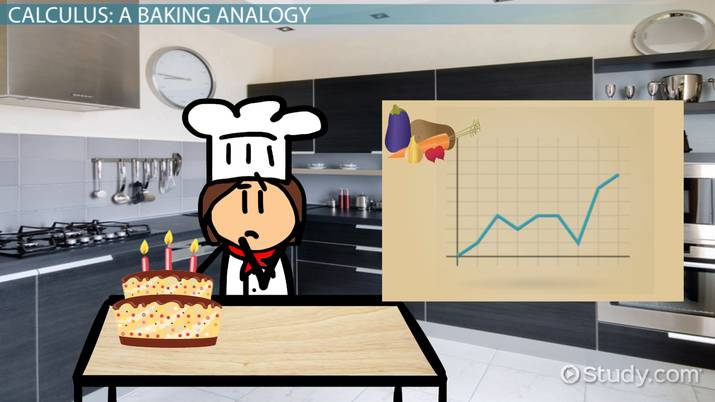An error occurred trying to load this video.

Try refreshing the page, or contact customer support.

Coming up next: Radians & Degrees on the CLEP Scientific Calculator

### You're on a roll. Keep up the good work!

Replay
Your next lesson will play in 10 seconds
• 0:00 Calculus: A Baking Analogy
• 0:46 Looking at Differentiation
• 3:46 Looking at Integration
• 5:41 Lesson Summary
Save Save

Want to watch this again later?

Timeline
Autoplay
Autoplay
Speed Speed

## Looking at Differentiation

Before we dive into formulas and rules for differentiation, let's look at some notation for differentiation. We can write the derivative of a function f(x) as: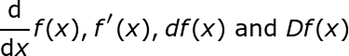We read these as: d by dx of f of x, f' prime of f of x, df of x and cap Df of x.

Although these and other notations are used for differentiation, we will use the d by dx and the prime notations in this lesson.

Here are some differentiation formulas: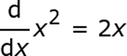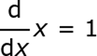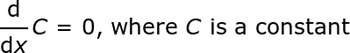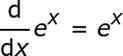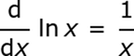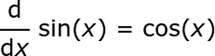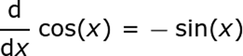You may have noticed in the first differentiation formula that there is an underlying rule. It's called the power rule, which says that the derivative of x raised to the power n is n times x raised to the power n minus 1.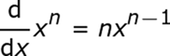This rule works even when n is not an integer. For example, the square root of x is x raised to the ½ power. Using the power rule for differentiation, we see how this works out in the example equation here: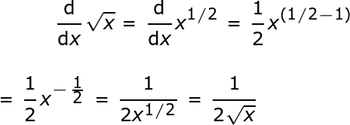As an example, let's show that the derivative of a constant is consistent with the power rule by noting that x^0 is equal to one. When we differentiate the constant C, we are in fact differentiating Cx^0. What you're looking at below is what we wanted to show: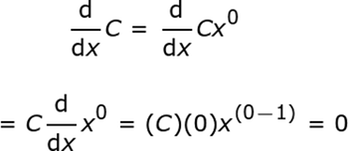In this last example, we used the coefficient rule, which states that the derivative of a constant times a function is that constant times the derivative of the function.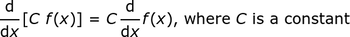Here are some other useful rules for differentiation, such as the product, quotient and chain rules. The product rule states that the derivative of the product of two functions is the derivative of the first function times the second function plus the first function times the derivative of the second function. As an example, check out the product rule in the action now: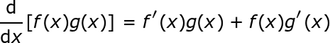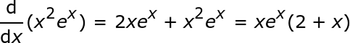There's also a way to find the derivative of one function divided by another function, the quotient rule, which says that the derivative of the quotient of two functions is the derivative of the numerator times the denominator minus the numerator times the derivative of the denominator all divided by the denominator squared. You can see this play out in the example below: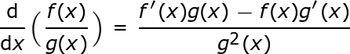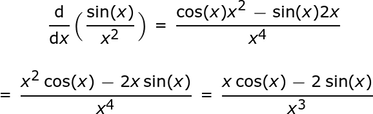To unlock this lesson you must be a Study.com Member.

### Register to view this lesson

Are you a student or a teacher?